Author: Oscar Cronquist Article last updated on March 17, 2022

## Count a specific text string in a cell

Question: How do I count how many times a text string exists in a cell value in Excel?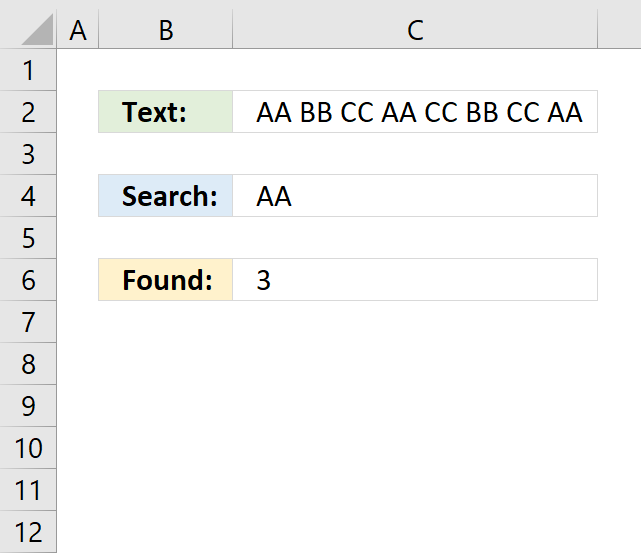The formula in cell C6 counts how many times a given text string is found in a cell value.

Formula in C6:

=(LEN(C2)-LEN(SUBSTITUTE(C2, C4, "")))/LEN(C4)

### Explaining formula in cell C6

#### Step 1 - Substitute given text string with nothing

SUBSTITUTE(C2, C4, "")

becomes

SUBSTITUTE("AA BB CC AA CC BB CC AA", "AA", "")

and returns " BB CC CC BB CC ".

#### Step 2 - Count text string characters

LEN(SUBSTITUTE(C2, C4, ""))

becomes

LEN(" BB CC CC BB CC ")

and returns 17.

#### Step 3 - Count text string characters in cell C2

LEN(C2)

becomes

LEN("AA BB CC AA CC BB CC AA")

and returns 23.

#### Step 4 - Subtract original character length with new text string character length

LEN(C2)-LEN(SUBSTITUTE(C2, C4, ""))

becomes

23 - 17

and returns 6.

#### Step 5 - Divide with search string character length

(LEN(C2)-LEN(SUBSTITUTE(C2, C4, "")))/LEN(C4)

becomes

6/LEN(C4)

becomes

6/2

and returns 3 in cell C6.

### Get Excel *.xlsx file

Count specific text string in a cell.xlsx

## 2. Count text string in a range (case sensitive)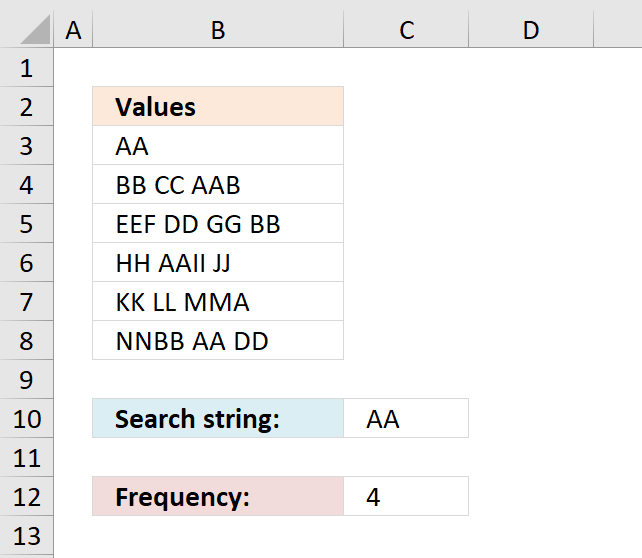### Question:

How do I count the number of times a text string exists in a column? The text string may exist multiple times in a cell, each instance is counted.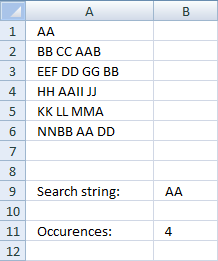### Array formula in cell B11:

=(SUM(LEN(tbl))-SUM(LEN(SUBSTITUTE(tbl, \$B\$9, ""))))/LEN(\$B\$9)

How to create an array formula

1. Copy array formula (Ctrl + c)
2. Select cell B11
3. Paste array formula (Ctrl + v) to formula bar4. Press and hold Ctrl + Shift
5. Press Enter
6. Release all keys

You can also use this formula to count how many times a specific character exists in a column in excel.

### Explain array formula in cell B11

=(SUM(LEN(tbl))-SUM(LEN(SUBSTITUTE(tbl, \$B\$9, ""))))/LEN(\$B\$9)

Step 1 - Replace existing text strings with new text string in named range tbl (A1:A6)

=(SUM(LEN(tbl))-SUM(LEN(SUBSTITUTE(tbl, \$B\$9, ""))))/LEN(\$B\$9)

Substitute(text, old_text, new_text, [instance_num]) replaces existing text with new text in a text string

SUBSTITUTE(tbl, \$B\$9, "")

becomes

SUBSTITUTE({"AA";"BB CC AAB";"EEF DD GG BB";"HH AAII JJ";"KK LL MMA";"NNBB AA DD"}, "AA", "")

returns this array:

{"";"BB CC B";"EEF DD GG BB";"HH II JJ";"KK LL MMA";"NNBB  DD"}

Step 2 - Return the number of characters in the named range tbl (A1:A6) without text string "AA"

=(SUM(LEN(tbl))-SUM(LEN(SUBSTITUTE(tbl, \$B\$9, ""))))/LEN(\$B\$9)

SUM(LEN(SUBSTITUTE(tbl, \$B\$9, "")))

becomes

SUM(LEN({"";"BB CC B";"EEF DD GG BB";"HH II JJ";"KK LL MMA";"NNBB  DD"}))

and returns 44

Step 3 - Return the number of characters in the named range tbl (A1:A6)

=(SUM(LEN(tbl))-SUM(LEN(SUBSTITUTE(tbl, \$B\$9, ""))))/LEN(\$B\$9)

SUM(LEN(tbl))

becomes

SUM(LEN({"AA";"BB CC AAB";"EEF DD GG BB";"HH AAII JJ";"KK LL MMA";"NNBB AA DD"}))

becomes

SUM({2;9;12;10;9;10})

returns 52.

Step 4 - Return the number of characters in cell B9

(SUM(LEN(tbl))-SUM(LEN(SUBSTITUTE(tbl, \$B\$9, ""))))/LEN(\$B\$9)

LEN(\$B\$9)

becomes

LEN("AA")

returns 2.

Step 5 - All together

=(SUM(LEN(tbl))-SUM(LEN(SUBSTITUTE(tbl, \$B\$9, ""))))/LEN(\$B\$9)

becomes

=(52-44)/2)

becomes

=8/2

returns 4.

Named ranges

tbl (A1:A6)

What is named ranges?

Get excel example file

count-text-string-in-a-column.xls

This blog article is one out of three articles on the same subject.

## 3. Count a given pattern in a cell value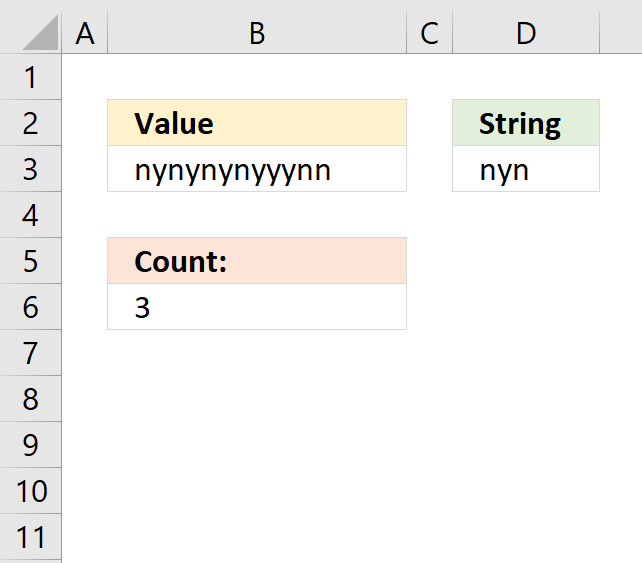The formula in cell B6 counts how many times the string (D3) is found in a cell value (B3) even if it overlaps another match.

Formula in cell B6:

=SUMPRODUCT(SIGN(MID(B3, ROW(A1:INDEX(A1:A1000, LEN(B3))), LEN(D3))=D3))

A regular count would result in 2 matches, see picture below.
You can find the formula here: Count specific text string in a cell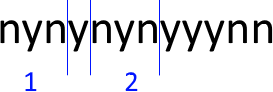A count where overlapping is allowed returns 3 matches and this is what is demonstrated in this article.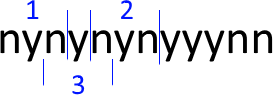### Explaining formula in cell B6

#### Step 1 - Build an array from 1 to the number of characters in the cell value

The LEN function counts the number of characters in cell B3.

LEN(B3) returns 13.

The INDEX function returns a cell reference based on a row number.

ROW(A1:INDEX(A1:A1000, LEN(B3)))

becomes

ROW(A1:INDEX(A1:A1000, 13))

becomes

ROW(A1:A13) and returns {1; 2; 3; 4; 5; 6; 7; 8; 9; 10; 11; 12; 13}

The ROW function returns the row number of a cell. If a cell range is used the ROW function returns an array of row numbers.

#### Step 2 - Extract all possible substrings from cell value

MID(B3, ROW(A1:INDEX(A1:A1000, LEN(B3))), LEN(D3))

becomes

MID(B3, {1; 2; 3; 4; 5; 6; 7; 8; 9; 10; 11; 12; 13}, LEN(D3))

becomes

MID("nynynynyyynn", {1; 2; 3; 4; 5; 6; 7; 8; 9; 10; 11; 12; 13}, 3)

and returns the following array: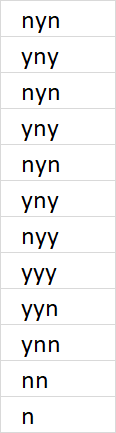#### Step 3 - Check if substring is equal to search string

MID(B3, ROW(A1:INDEX(A1:A1000, LEN(B3))), LEN(D3))=D3

becomes

{"nyn"; "yny"; "nyn"; "yny"; "nyn"; "yny"; "nyy"; "yyy"; "yyn"; "ynn"; "nn "; "n "; " "}=D3

and returns {TRUE; FALSE; TRUE; FALSE; TRUE; FALSE; FALSE; FALSE; FALSE; FALSE; FALSE; FALSE; FALSE}.

#### Step 4 - Convert boolean values to the corresponding number

The SUMPRODUCT function can't handle boolean values so the SIGN function converts them into numbers. TRUE = 1 and FALSE = 0.

SIGN(MID(B3, ROW(A1:INDEX(A1:A1000, LEN(B3))), LEN(D3))=D3)

becomes SIGN({TRUE; FALSE; TRUE; FALSE; TRUE; FALSE; FALSE; FALSE; FALSE; FALSE; FALSE; FALSE; FALSE})

and returns {1; 0; 1; 0; 1; 0; 0; 0; 0; 0; 0; 0; 0}.

#### Step 5 - Count values in array

SUMPRODUCT(SIGN(MID(B3, ROW(A1:INDEX(A1:A1000, LEN(B3))), LEN(D3))=D3))

becomes

SUMPRODUCT({1; 0; 1; 0; 1; 0; 0; 0; 0; 0; 0; 0; 0})

and returns 3 in cell B6. 1 + 0 + 1 + 0 + 1 + 0 + 0 + 0 + 0 + 0 + 0 + 0 + 0 = 3.

### Get Excel *.xlsx file

Count overlapping text string in a cell.xlsx